Courses

# Summary - Friction of Rigid Bodies Mechanical Engineering Notes | EduRev

## Mechanical Engineering : Summary - Friction of Rigid Bodies Mechanical Engineering Notes | EduRev

The document Summary - Friction of Rigid Bodies Mechanical Engineering Notes | EduRev is a part of the Mechanical Engineering Course Engineering Mechanics - Notes, Videos, MCQs & PPTs.
All you need of Mechanical Engineering at this link: Mechanical Engineering

A. FRICTION

In the preceding chapters we have usually assumed that the forces of action and reaction between contacting surfaces act normal to the surfaces. This assumption is valid only between smooth surfaces.
No surface is perfectly smooth, a surface contains microscopic “bumps”. When two surfaces are moving against each other, these bumps interlock against each other and oppose the motion. Friction originates from this opposition.

 Types of Friction (a) Dry Friction : Dry friction occurs when the unlubricated surfaces of two solids are in contact under a condition of sliding or a tendency to slide.(b) Fluid Friction : Fluid friction occurs when adjacent layers in a fluid (liquid or gas) are moving at different velocities.(c) Internal Friction : Internal friction occurs in all solid materials which are subjected to cyclical loading. For highly elastic materials the recovery from deformation occurs with very little loss of energy due to internal friction.

Types of Dry Friction

1. Static Friction : It is the friction experienced by a body when it is at rest or on the verge of motion. Eg – Body resting on an ramp with small inclination

2. Dynamic Friction : It is the friction experienced by a body when it is in motion. Eg – Resisting force experienced by a rolling skater

 Static Friction Dynamic Friction 1. Static friction is the frictional force acting between two surfaces which are attempting to move, but are not moving. The body remains in equilibrium2. Static friction is proportional to the external forces and increases linearly with the force applied until it reaches a maximum value.3. Static friction could have a value less or greater than the value for kinetic friction. But it cannot increase beyond a maximum value called the limiting value of frictional force.4. The coefficient of static friction is less than the dynamic friction5. Eg : Body resting on an ramp with small inclination 1 Kinetic friction is the frictional force acting between two surfaces which are in motion against each other2. Kinetic friction remains constant regardless of the force applied. It is independent of mass and acceleration.3. Kinetic friction has a value less than the limiting value of static friction. 5. Eg : Resisting force experienced by a rolling skater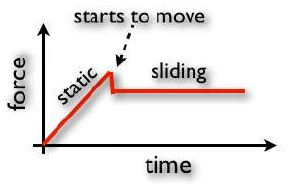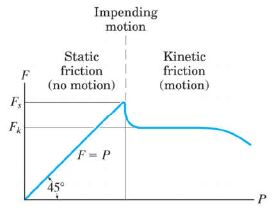Laws of Coloumb’s Friction (Dry Friction)

1. When two bodies are in contact, the direction of force of friction on one of them is opposite to the direction in which this body has a tendency to move tangent to the surface.

2. The frictional force is independent of the area of contact of the surfaces.

3. The force of friction is dependent upon the types of materials of the two bodies in contact.

4. The limiting frictional force bears a constant ratio with the normal reaction.

5. When one body is just on the verge of sliding over another body, the force of friction is maximum and this maximum frictional force is called Limiting Static Frictional Force

6. Limiting static frictional force is greater than kinetic frictional force for any two surfaces of contact.

7. The kinetic frictional force is independent of the relative velocities of the bodies in contact.

Limiting Force of Friction

The maximum value of frictional force at the surfaces of contact when the body is at the verge of motion is called limiting static frictional force (Fs).

When this value is reached, the block is in unstable equilibrium since any further increase in P will cause the block to move. It is experimentally found that friction directly varies as the applied force until the movement produces in the body.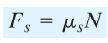Where Fs = Limiting static frictional force
µs = coefficient of static friction
N = Normal reaction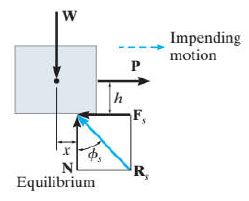Coefficient of static friction

It is defined as the ratio of limiting force of friction (Fs) to the normal reaction (N) between the two bodies. It is constant denoted by µs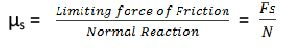Angle of friction

It is defined as the angle made by the resultant of the normal reaction and frictional force (R) to the normal reaction (N). It is denoted by φ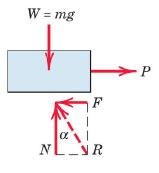Cone of friction

It is defined as the right circular cone with vertex at the point of contact of the two surfaces, axis in the direction of the normal reaction (N) and semi-vertical angle equal to the angle of fiction φ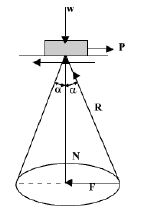Angle of repose

It is defined as the maximum inclination of a plane at which a body remains in equilibrium over the inclined plane by assistance of friction alone.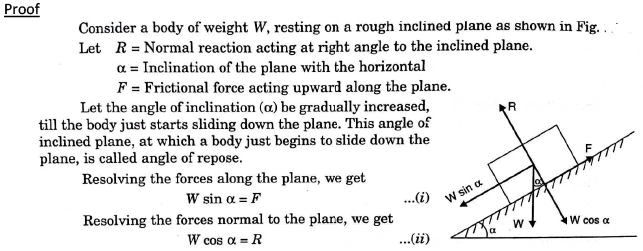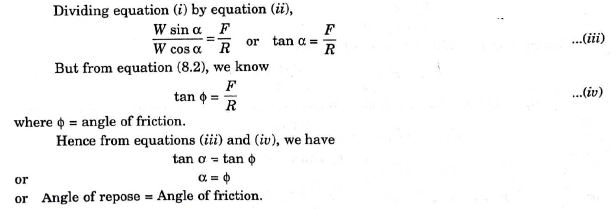Offer running on EduRev: Apply code STAYHOME200 to get INR 200 off on our premium plan EduRev Infinity!

,

,

,

,

,

,

,

,

,

,

,

,

,

,

,

,

,

,

,

,

,

;